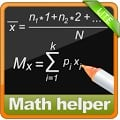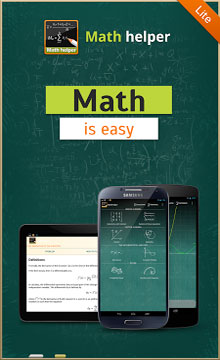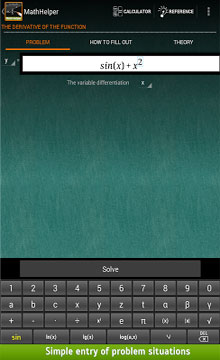# Math Helper Lite – Algebra APKMath Helper Free is the best application on the market, which solves mathematical problems and shows step-by-step solution.
It’s easy – you enter a problem and get the answer, detailed solution and a theory reference.
THIS IS ORIGINAL MATH HELPER. Avoid cheap clones of this app on the market and see no further – download it right now!
***
OUR USERS SAY:
«This is amazing app this helped me to study easily without any doubt.. Without any guidence i can get full marks in maths … Love this app 🙂 🙂 :D» – Pulkit G.
***
WHAT IT HAS
– Linear Algebra – Operations with matrices (solving matrix)
– Linear Algebra – Solving systems of linear equation (from 2 to 10 equation at a time)
– Vector Algebra – Vector (vector problems)
– Vector Algebra – Geometry Shapes (geometry – triangles, pyramids, planes)
– Calculus – Derivative (Derivative of the function, of a function defined parametrically, of an implicit one)
– Calculus – Indefinite Integral (integral solver, antiderivatives)
– Limits (limits calculator solver)
– The theory of probability (probability theory, statistics)
– Calculus – The number and sequence (prime factorization, lcm and gcd, complex numbers, arithmetic and geometric progressions and numbers, Diophantine equation, Euler function)
– Function plotter (interactive graph plotter)
– Also contains great scientific calculator and theoretical handbook
Math Helper Free is an universal assistant app for step-by-step solving mathematical problems for Algebra 1-2, Calculus (integral, Derivative, antiderivatives, vector, matrices (matrix), limits, equation, shapes, number etc) for school, secondary, college and university students and everyone who learn.
It allows you not only to see the answer or result of a task, but also a detailed step-by-step solution.
Also great feature is built-in scientific calculator and reference handbook – much easier to learn.
***
FEATURES AND ACHIEVEMENTS
. 10 topics and 60+ sub-section.
. Localization for Russian, English, Italian, French, German and Portuguese
. Intel ® Learn Series Alliance quality mark
. EAS ® approved
. More than 10’000 customers all over the world supported development of Math Helper Free by doing purchase
. The application is equipped with a convenient multi function calculator and extensive theoretical reference handbook to learn better
Derivative, antiderivatives, limits, geometric shapes, the task of statistics, probability theory, matrices or matrix (large spectrum of tasks with matrices (matrix) supported), systems of equation and other mathematical solving – this and more in Math Helper!
***
SUPPORT
. Thank you all helping us to reach 800’000+ downloads of Lite version
. Or you could reach us directly by email
***
WHAT IS NEXT
We have plans to implement
. Numbers and polynomial solving, division and multiplication
. Fractions and definite integral (antiderivatives)
. Double integral (antiderivatives)
. More tasks on geometry, plotter
. Limits step-by-step
. New applications, like Calculus symbolic calculator, Physics Solver, Physics Ref, Chemistry Solver, Geometry Shapes Solver and Plotter, theoretical reference handbook app.
***
Having problems with Probability theory, geometry shapes solution, integral, matrices (matrix), graph plotter, even more extensive theoretical reference handbook and much much more? Want to learn better? See no further – download this application right now!
P.S. Support us by liking us on Facebook
P.P.S Full solution is available not for all problem types, integral and limit problems available only in Full version.
******** THANK YOU!!! ********

Size : 20M
Current Version : 3.1.4
Requires Android : 2.3.3 and up